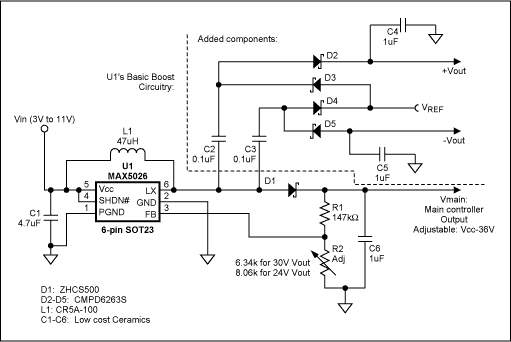# Tracking Power Supply Has Dual Outputs \r\n

Designing a stable bipolar supply for powering op-amps, multiplexers, switches, etc. can be difficult, especially if the two voltages must track each other with respect to a non-zero or adjustable reference level. Such a regulated supply for low-power applications (Figure 1) produces a main-controller output voltage (VMAIN) and two tracking voltages symmetric about an adjustable reference voltage (VREF). You create the circuit by adding four Schottky diodes (D2–D5) and two flying capacitors (C2–C3) to the basic boost-converter circuit for U1.Figure 1. This single-IC circuit generates the bipolar voltages required in many industrial analog applications, as well as contrast-control voltages for an STN LCD.

U1 is an efficient, single-output boost converter for applications requiring outputs up to 36V and a wide input-voltage range (3V to 11V). U1 requires no external switching devices and draws a typical supply current of only 350µA, making it ideal for handheld and point-load applications. It is characterized for loads up to 120mW.

The ±30V outputs are centered about a reference level of VREF = 0V (Figure 2). For balanced loads of 0.5mA to 2mA, tracking is excellent over a wide input range. Figure 3 shows how +VOUT and -VOUT track each other as VREF is moved away from 0V. One example of the need for a non-zero VREF is indicated in Figure 4, in which the LCD-contrast voltages must be symmetric about VREF to avoid a DC component across the liquid crystal, which in turn can damage the LCD or shorten its life.Figure 2. This graph shows the Figure 2 outputs of ±VOUT and VMAIN across the full 3V–11V input voltage range, under varying load conditions.Figure 3. This graph shows that the ±VOUT outputs in Figure 1 track each other with respect to changes in the reference voltage: ±VOUT = VREF ± VMAIN.Figure 4. To avoid a damaging DC component across the LCD, these contrast waveforms are symmetrical about the reference level VREF.

A FET internal to U1 repeatedly connects LX (pin 6) to ground and then releases it, causing the LX voltage to toggle between ground and VMAIN plus one diode drop (D1). That action generates the ±VOUT voltages as follows:

-VOUT output, phase 1: The rise of LX voltage to VOUT + VDIODE forces voltage on the other side of C3 to VREF + VDIODE, creating a differential of VMAIN -VREF across C3. The LX node is our reference point. Phase 2: As LX is switched to ground, the load side (-VOUT) sees -VMAIN + VREF, forcing current from the -VOUT load through D5, and the cycle repeats itself. Note that +VOUT and -VOUT develop on alternate phases. The resulting -VOUT voltage is

-VOUT = -VMAIN + VREF + VDIODE.

+VOUT output, phase 2: When LX is switched to ground, the load side of C2 sees VREF - VDIODE. Then, (phase 1) the rise of LX to VMAIN + VDIODE forces a voltage of VMAIN + VREF on the other side of C2. The resulting +VOUT voltage is:

+VOUT = VMAIN + VREF - VDIODE.

These load equations suggest, and Figures 2 and 3 illustrate, that -VOUT and +VOUT track each other with respect to VMAIN, and are offset by one diode drop from VREF. D1–D5 are low-current Schottky diodes. C2 and C3 can be ceramic capacitors in the range 1nF to 100nF, preferably with voltage ratings of approximately 2 × |VOUT|. Larger values of C2 and C3 provide more stable outputs under a wide range of load currents. L1 is typically 47µH, and the output capacitors C4–C6 (shown with 1µF values) may be sized according to the allowable output ripple.

A similar version of this article appeared in the July 15, 2002 issue of Planet Analog magazine.